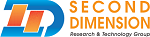SecondBASIC Documentation v3

## User Defined Routines

What is a User Defined Routine? It's a routine that you define using the Declare statement that executes code when called. There are 2 types of Declare statements that can be written; one for a sub-routine and one for functions.

What is the difference between a sub-routine and a function? A function must have an output, while a sub-routine just performs the specific tasks. A sub-routine cannot have an output. Both types of routines can have inputs, though it's not required.

Subs and functions do a few things: it allows you to organize your code, it allows you to create your own "commands and functions", and it lets you reuse code without having to write the same code over and over. You'd use a function whenever you need a return value from the executed code, otherwise you'd use a sub-routine. With a sub-routine, you'd just reference it by name to execute the code. A function requires an assignment or immediate usage to be called.

Let's get moving onto an example of creating a sub-routine. In the example below, 2 sub-routines will be defined: one with arguments and one without. This will let you see how to define them and call them.

HelloSub
AltPrint "Hello World",10,5
End

Declare Sub AltPrint(text\$ As String, x As Integer, y As Integer)
ASCIIStart = 0 ' Where the ASCII table is loaded in VRAM
For i = 0 To Len(text\$)
char\$ = Mid\$(text\$,i,1)
ascii = Asc(char\$)+ASCIIStart
ascii+=ASCIIStart
DrawTile ascii,x,y
x++
Next
End Sub

Declare Sub HelloSub()
Print "Hello Sub!"
End Sub

In the above example, take notice that the order of the declarations and usage does not matter. Aside from that, subs are just routines that you'll commonly use throughout your program. It may range from resetting all variables to drawing tiles, and so on - really whatever you need to do more than once.

Functions operate similarly to sub-routines with the exception that they return a value of some sort. Let's see how to declare and use a function:

a = Exponent(2,3)
Print a
Print Exponent(4,3)

End

Declare Function Exponent(x As Integer, y As Integer) As Integer
ex = x ^ y
Return ex
'Return x^y
End Function

The declaration is almost identical, except you have to specify a data type at the end of the argument list.